ECMAScript 2016

# ECMAScript 2016

ECMAScript 2016

In this tutorial, you will learn about ECMAScript 2016 or ES 2016:-
Introducing the new features that ECMAScript 2016 (ES7) adds to JavaScript :-

• JavaScript Exponentiation (**)
• JavaScript Exponentiation assignment (**=)
• JavaScript String includes() Method

JavaScript Exponentiation Operator

Gives the same result as an Exponentiation operator like Math.pow(a, b).

The Syntax of the Exponentiation Operator is as follows: -
Math.pow(10, 2) 🡺 10 ** 2

Example 1 –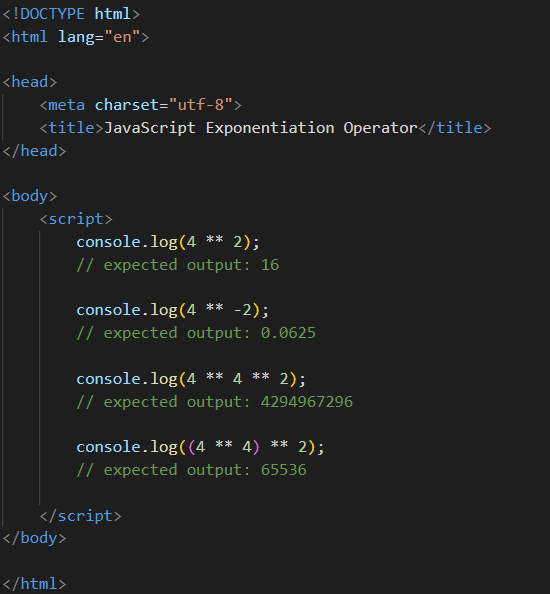Output –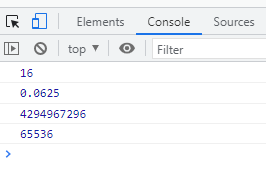JavaScript Exponentiation assignment

The exponentiation assignment operator (**=) raises the value of a variable to the power of the right operand.

Example 1 –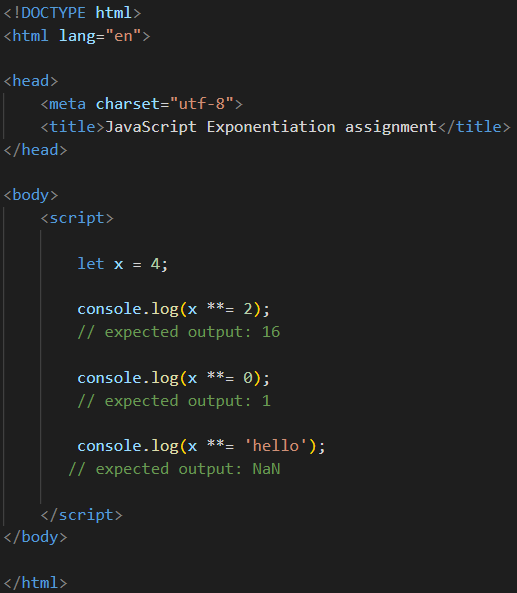Output –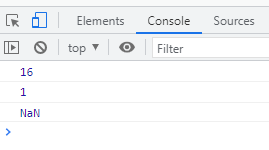JavaScript String includes() method

In this Tutorial, we will learn about JavaScript String includes() method with the help of following below:
The includes() method will be true if the string includes the specified word else return false.
Syntax
var includesStr = str.includes(searchString, position);

Example 1 –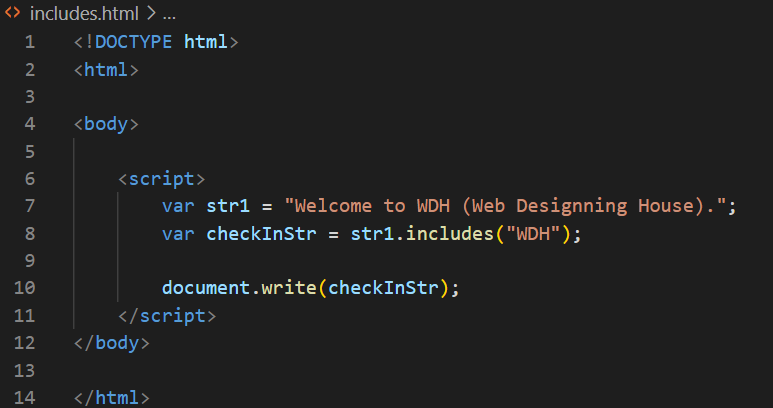Output -
true

Example 2 –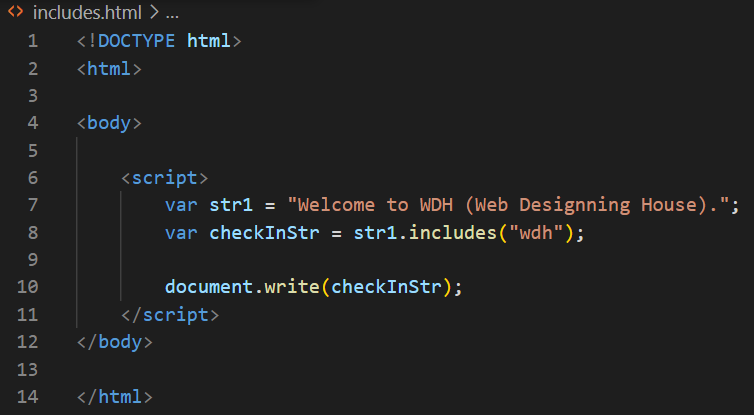Output –

false